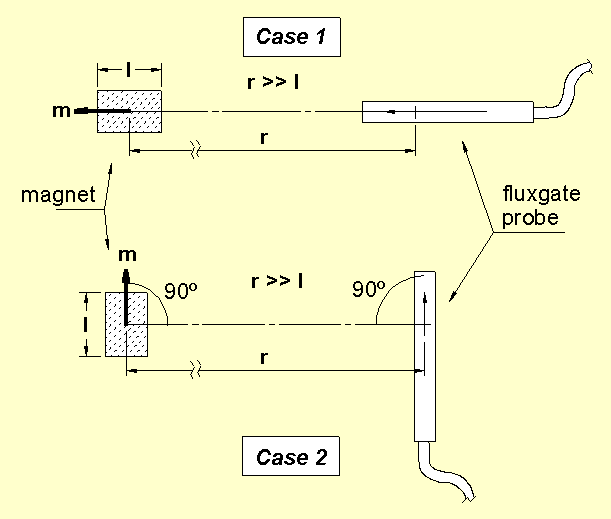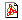Serviciencia, S.L. - Application Note: NAS01-i

Magnetic Moment easy measurement

Normally, Helmholtz coils connected to an integrator are used for this measurement on one magnet, taking also into account the magnet geometry. Besides this, standardised magnets are often needed to calibrate the system. Due to all this, it results in a quite cumbersome procedure, especially when an occasional, single, measurement is demanded.

This is a simple procedure to measure the Magnetic Moment (m) on one magnet, or one magnet array, by using one high sensitivity magnetometer. The error of the obtained Moment (m) may be easily below ±5 %. The measurement does not depend on the shape or homogeneity of the magnet under test. The "magnet under test" can be almost any magnetised object, as one machine, an engine, or even a satellite, for example, mounted on a rotating table.Case 1: m = 5 · B · r3 m = Magnetic Moment, in A.m2. B = measured field, in µT. r = magnet-to-probe distance, in metres. Case 2: m = 10 · B · r3

In Case 1, on which the magnet and the probe are coaxial, sensitivity is double than in Case 2, from what Case 1 results the more adequate configuration when it is possible to be used.

The distance (r) must be about 10 times the bigger magnet dimension. In such a way the measurement will be practically independent from the magnet shape and homogeneity. One distance of 5 times could give satisfactory results.

To improve the measurement and dismiss the Earth magnetic field, it is convenient to perform two measurements with the magnet 180º rotated in respective of each. Then, one reading is subtracted from the other and the result is divided by 2 to get B.

EXAMPLE: Be one bar magnet of 5 cm in length, with one Moment (m) = 1 A.m2, arranged as in Case 1, with r = 0.5 m. The measured field will be B = 1.6 µT. If it were r = 0.25 m, then it would be B = 12.8 µT, approximately.

The magnetometer Bartington Mag-01H, with a resolution of 0.1 nT, is very well suited for that kind of measurements. Also the Mag-01, with 1 nT resolution, can be used in many cases, as well as one Bartington Mag-01 or Mag-03 probe.

 PDF version of this document (click here):Updated: 21/10/03